Author: Oscar Cronquist Article last updated on October 31, 2018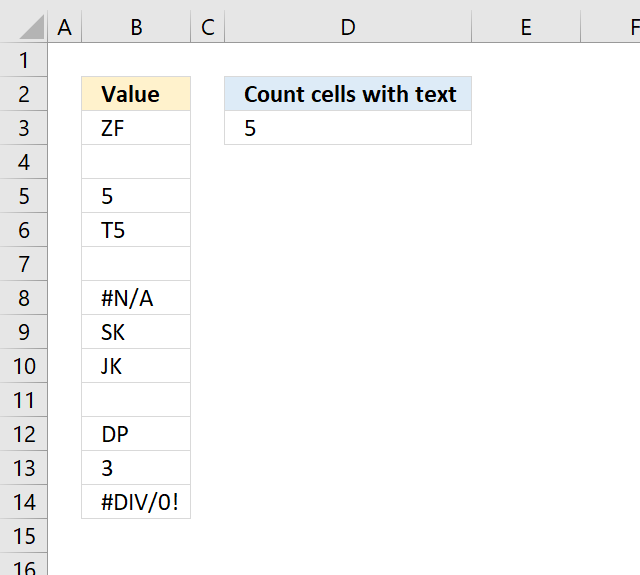The following formula in cell D3 counts cells with values stored as text.

=SUMPRODUCT(ISTEXT(B3:B14)*1)

In other words, cells containing nothing, errors, boolean values, and numbers are not counted.

Numbers stored as text are counted, as well as cells containing a space a character or more.

#### Explaining formula

Step 1  - Identify values stored as text

The ISTEXT function returns TRUE or FALSE depending on if a cell has a value stored as text.

ISTEXT(B3:B14) returns {TRUE; FALSE; FALSE  ... }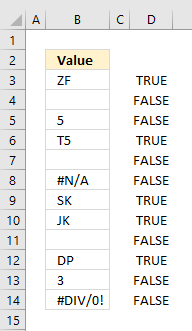Step 2 - Convert boolean values to numbers

To count boolean values (TRUE, FALSE) we need to convert them into numbers. TRUE = 1 and FALSE = 0 (zero).

ISTEXT(B3:B14)*1 becomes {TRUE; FALSE; FALSE  ... }*1

and returns {1; 0; 0; ... }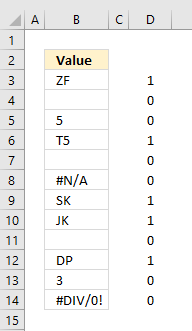Step 3 - Count numbers

The SUMPRODUCT function has a great advantage over the SUM function, in most cases, you don't need to enter the formula as an array formula if you are working with arrays.

SUMPRODUCT(ISTEXT(B3:B14)*1)

becomes

SUMPRODUCT({1;0;0;1;0;0;1;1;0;1;0;0})

and returns 5 in cell D3. 1+0+0+1+0+0+1+1+0+1+0+0 = 5.

### Count cells with text excluding cells containing a space character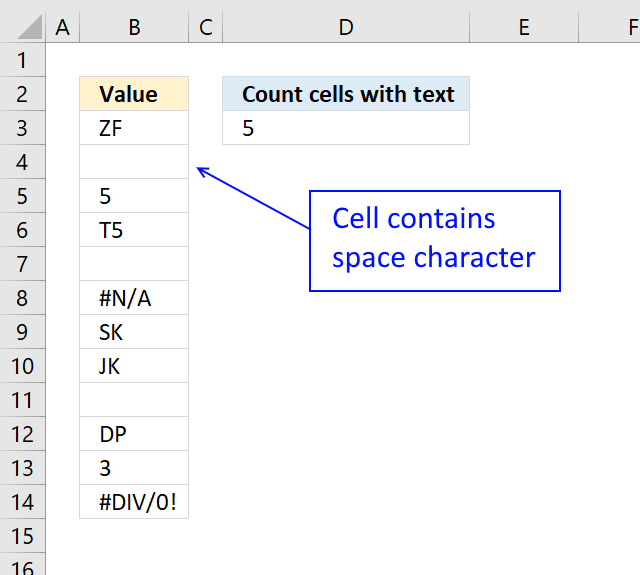Cell B4 contains a space character, the array formula below does not count cell containing a space character.

=SUM(IF(ISTEXT(B3:B14),(B3:B14<>" "),0)*1)

B3:B14<>" "  makes sure that cells containing a space character are not counted.

To enter an array formula, type the formula in a cell then press and hold CTRL + SHIFT simultaneously, now press Enter once. Release all keys.

The formula bar now shows the formula with a beginning and ending curly bracket telling you that you entered the formula successfully. Don't enter the curly brackets yourself.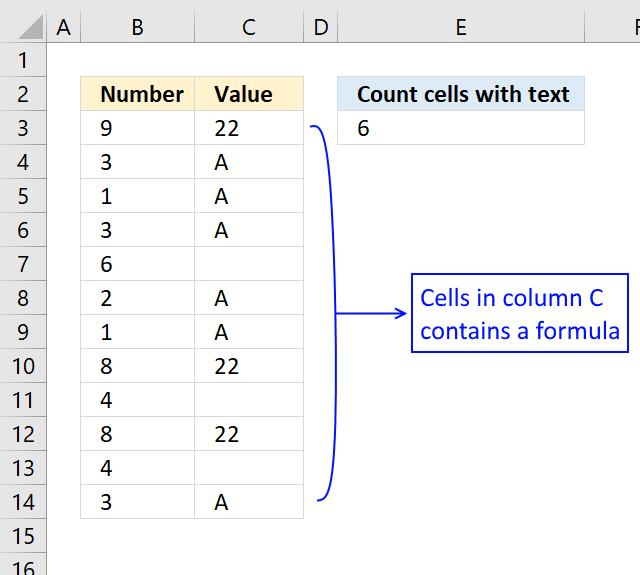The formula below lets you count text values returned from an Excel function.

=SUMPRODUCT(ISTEXT(C3:C14)*(C3:C14<>""))

The ISTEXT function handles empty values from an Excel function as a text value. To avoid counting those non-values I simply use the smaller than and larger than signs <> meaning not equal to.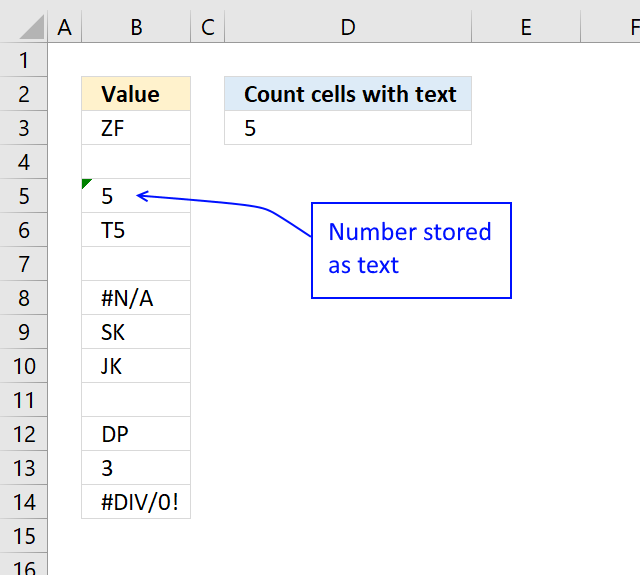Cell B5 contains a number stored as text, to exclude that number use the following formula:

=SUMPRODUCT(ISTEXT(B3:B14)*NOT(ISNUMBER(B3:B14*1)))